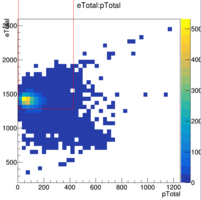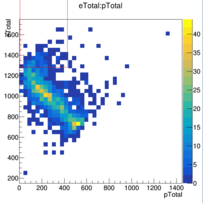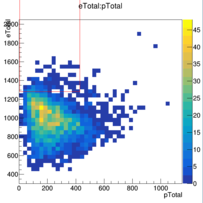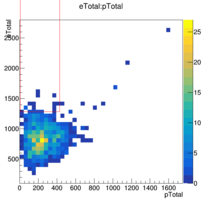# Strange cut values from TMVA Rectangular Cuts classificator

I am using TMVA Rectangular Cuts classificator.
After training I get this cut values:

``````Cuts                     : Cut values for requested signal efficiency: 0.9
: Corresponding background efficiency       : 0.044991
: Transformation applied to input variables : None
: ------------------------------------------
: Cut[ 0]:    1281.78 < eTotal <=    3368.63
: Cut[ 1]:    4.28152 < pTotal <=    428.097
: ------------------------------------------
``````

Look at my training trees (red “rectangle” illustrates cut values):The quesion is:

Where so high (3368 MeV) upper limit on `eTotal` comes from taking into account there is no, even nearly, such values of `eTotal` in the training trees.

Hi,

Sorry for the late reply. I think I saw from a different question that you are using the genetic fitter. If that’s the case then basically any upper limit that is larger than your training data would be valid.

The genetic algorithm would just pick one value that is large enough and there would not be any incitement for improving it.

Cheers,
Kim

See this post, please. There I show that even if I use `CutRange` options the limits too high.

Could you provide a minimal example with some random input data that reproduce the problem? That would be very helpful.

This could be useful if you could:

``````TTree * createData(Double_t mu, Double_t sigma)
{
Double_t x;
Double_t y;

Int_t N_POINTS = 1000;

TRandom3 rng;

static int i = 0;
TTree * data = new TTree();
data->Branch("x" , &x, "x/D");
data->Branch("y" , &y, "x/D");

for (Int_t n = 0; n < N_POINTS; ++n) {
x = rng.Gaus(mu, sigma);
y = rng.Gaus(mu, sigma)
data->Fill();
}

return tree;
}
``````

Cheers,
Kim

It might be I did not understand you. But here are all necessary files to produce errors. Program and Training trees. Just run it

``````root TMVAClassification.C
``````